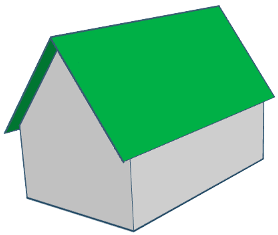# Roof Pitch Calculator

#### What is the Roof Pitch?

Commonly, the pitch is known as the slope (incline) of the roof.
Roof pitch can also be defined as the vertical rise divided by its horizontal span (or run).
In construction, the roof pitch can be known as stair while in trigonometry it is known as the tangent function.

Pitch is expressed as a degree or percentage in countries that use the metric system while in countries that use imperial measurement, pitch is expressed with rise first and run second. In US, instead of the whole run, 12 inch is used and the pitch is how much rise or fall is for 12 inch of run.

#### Calculate Roof Pitch from Rise and Run #*All fields are required:
Run
Rise
Pitch angle (degrees): =
Roof pitch (x:12): =
Rafter Length: =
* For decimal dimensions, use the dot notation! (.)

### More Roof Pitch Calculators

Angle

#### Calculate degrees from pitch #

Pitch (x:12):#
Posts are reviewed by an admin beofore showing on the website.
Pat Foisey
when Calculating the Roof Pitch from Rise and Run. your form should give the answer for rafter lenth othherwise i like this site.